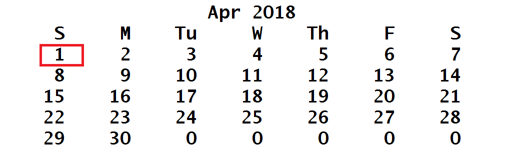# Easter, Revisited

Today is Easter Sunday. Why? How is the date of Easter determined?This is a revision of a blog post from 2013.

### Contents

#### Easter Day

Easter Day is one of the most important events in the Christian calendar. It is also one of the most mathematically elusive. In fact, regularization of the observance of Easter was one of the primary motivations for calendar reform centuries ago.

Easter is linked to the Jewish Passover. The informal rule is that Easter Day is the first Sunday after the first full moon after the vernal equinox. But the ecclesiastical full moon and equinox involved in this rule are not always the same as the corresponding astronomical events, which, after all, depend upon the location of the observer on the earth.

I am using a revision of the easter program in my blog post from 2013 and Experiments with MATLAB. Let's check this year.

   easter_2018 = datestr(easter(2018))

easter_2018 =
'01-Apr-2018'


#### Don Knuth

My MATLAB® program is based on the algorithm presented in the first volume of the classic series by Donald Knuth, The Art of Computer Programming. Knuth has used it in several publications to illustrate different programming languages. The task has often been the topic of an exercise in computer programming courses.

Knuth says that the algorithm is due to the Neapolitan astronomer Aloysius Lilius and the German Jesuit mathematician Christopher Clavious in the late 16th century and that it is used by most Western churches to determine the date of Easter Sunday for any year after 1582.

The date varies between March 22 and April 25.

#### Metonic cycle

The earth's orbit around the sun and the moon's orbit around the earth are not in sync. It takes the earth about 365.2425 days to orbit the sun. This is known as a tropical year. The moon's orbit around the earth is complicated, but an average orbit takes about 29.53 days. This is known as a synodic month. The fraction

year = 365.2425;
month = 29.53;
format rat
ratio = year/month

ratio =
6444/521


is not the ratio of small integers. However, in the 5th century BC, an astronomer from Athens named Meton observed that the ratio is very close to 235/19.

format short
ratio
meton = 235/19

ratio =
12.3685
meton =
12.3684


In other words, 19 tropical years is close to 235 synodic months. This Metonic cycle was the basis for the Greek calendar and is the key to the algorithm for determining Easter.

#### MATLAB program

Here is the revised MATLAB program. Try other years.

Can you spot the change made to my old program in the blog post from 2013?

type easter

function dn = easter(y)
% EASTER  Date of Easter.
% EASTER(y) is the datenum of Easter in year y.
% Example:
%   datestr(easter(2020))
%
% Ref: Donald Knuth, The Art of Computer Programming,
%      Fundamental Algorithms, pp. 155-156.

%   Copyright 2014-18 The MathWorks, Inc.

% Golden number in 19-year Metonic cycle.
g = mod(y,19) + 1;

% Century number.
c = floor(y/100) + 1;

% Corrections for leap years and moon's orbit.
x = floor(3*c/4) - 12;
z = floor((8*c+5)/25) - 5;

% Epact.
e = mod(11*g+20+z-x,30);
if (e==25 && g>11 || e==24), e = e + 1; end

% Full moon.
n = 44 - e;
if n < 21, n = n + 30; end

% Find a Sunday.
d = floor(5*y/4) - x - 10;

% Easter is a Sunday in March or April.
d = n + 7 - mod(d+n,7);
dn = datenum(y,3,32);


#### References

 Donald E. Knuth, The Art of Computer Programming, Volume 1: Fundamental Algorithms (3rd edition), pp. 159-160, Addison-Wesley, 1997, ISBN 0-201-89683-4, PDF available.

 Wikipedia, Primary article on Easter. <http://en.wikipedia.org/wiki/Easter>

 Wikipedia, Computus, details on calculation of Easter. <http://en.wikipedia.org/wiki/Computus>

 Wikipedia, Metonic cycle. <http://en.wikipedia.org/wiki/Metonic_cycle>

Published with MATLAB® R2018a

|